# 菲涅尔反射 Fresnel Reflectance

$\mathbf{r}_i = 2(\mathbf{n}\cdot\mathbf{l})\mathbf{n}-\mathbf{l}$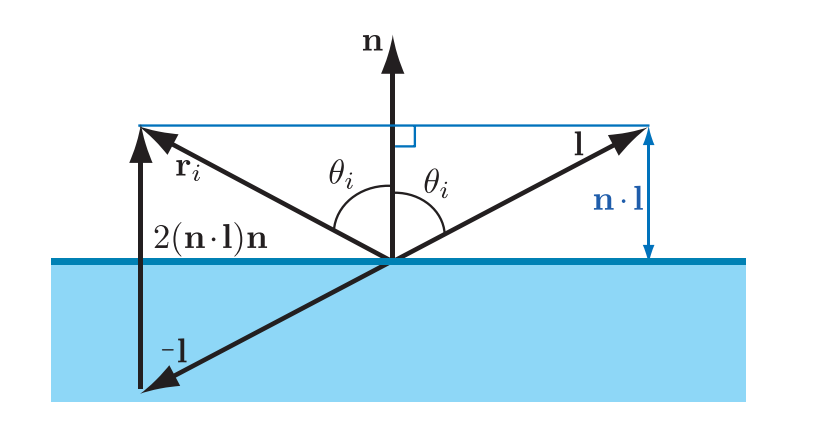## 菲涅尔现象 Fresnel Effect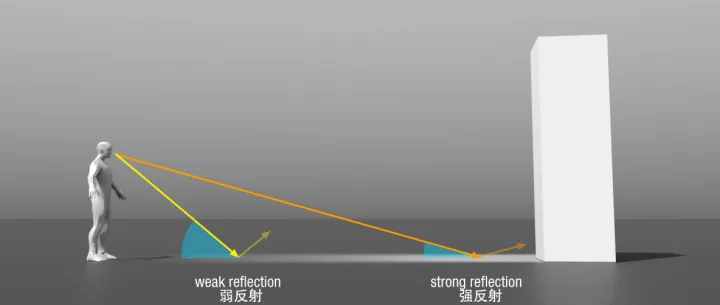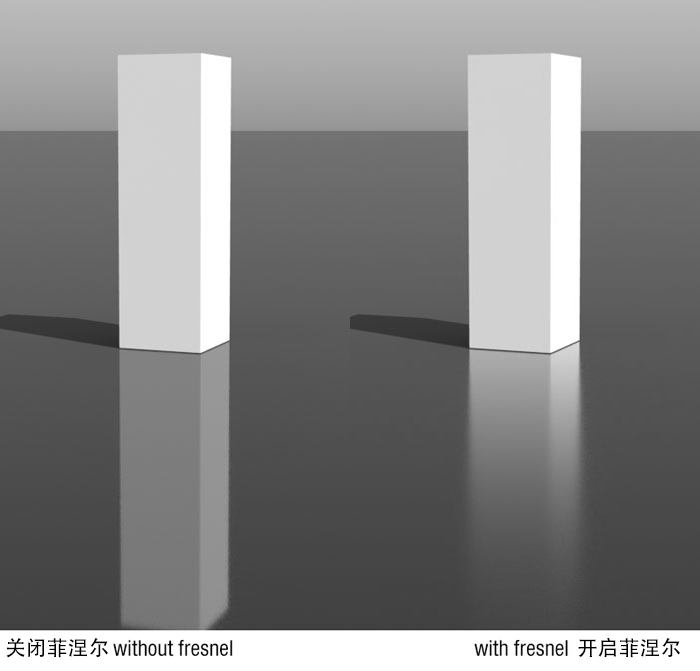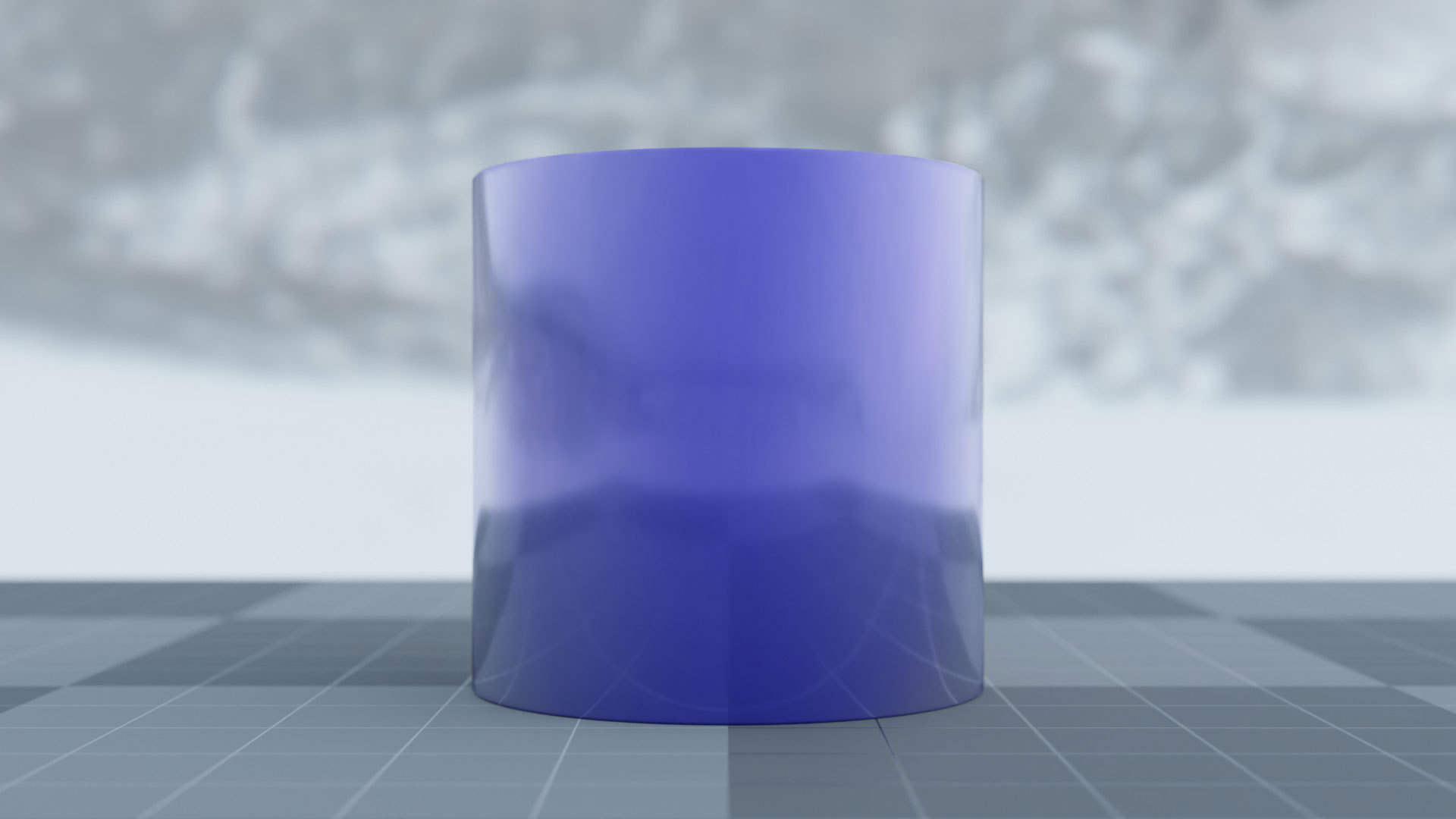## 外反射 External Reflection

$$n_1<n_2$$，即光从折射率较小的一边射到折射率较大的一边时发生的反射我们称为外反射，反之称为内反射。物体外面通常是空气，空气的折射率约是 $$1.003$$，为了简便，我们认为 $$n_1=1$$

• $$\theta_i=0^\circ$$，即垂直入射时，$$F_0$$ 可以被认为是该物质的镜面反射颜色；
• $$\theta_i$$ 逐渐增大时，$$F(\theta_i)$$ 也逐渐增大，并且对于所有频率的光，当 $$\theta_i$$ 达到 $$90^\circ$$ 时，$$F(\theta_i)=1$$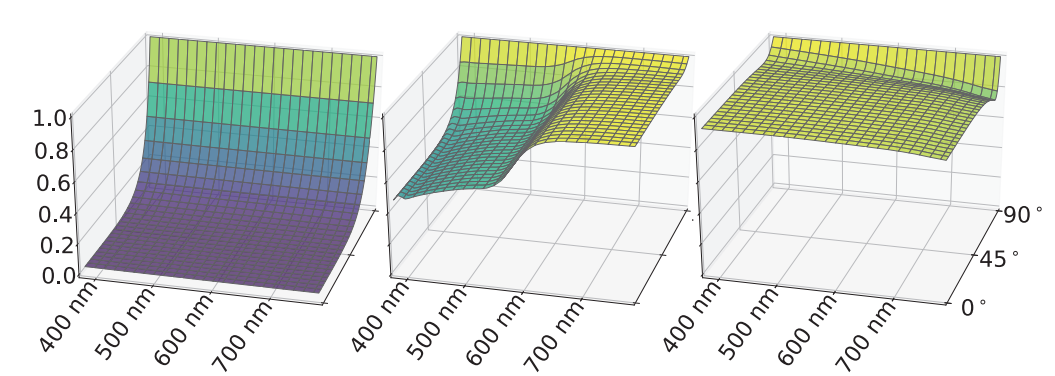$F(\mathbf{n},\mathbf{l}) \approx F_0 + (1 - F_0)(1-(\mathbf{n}\cdot \mathbf{l})^+)^5$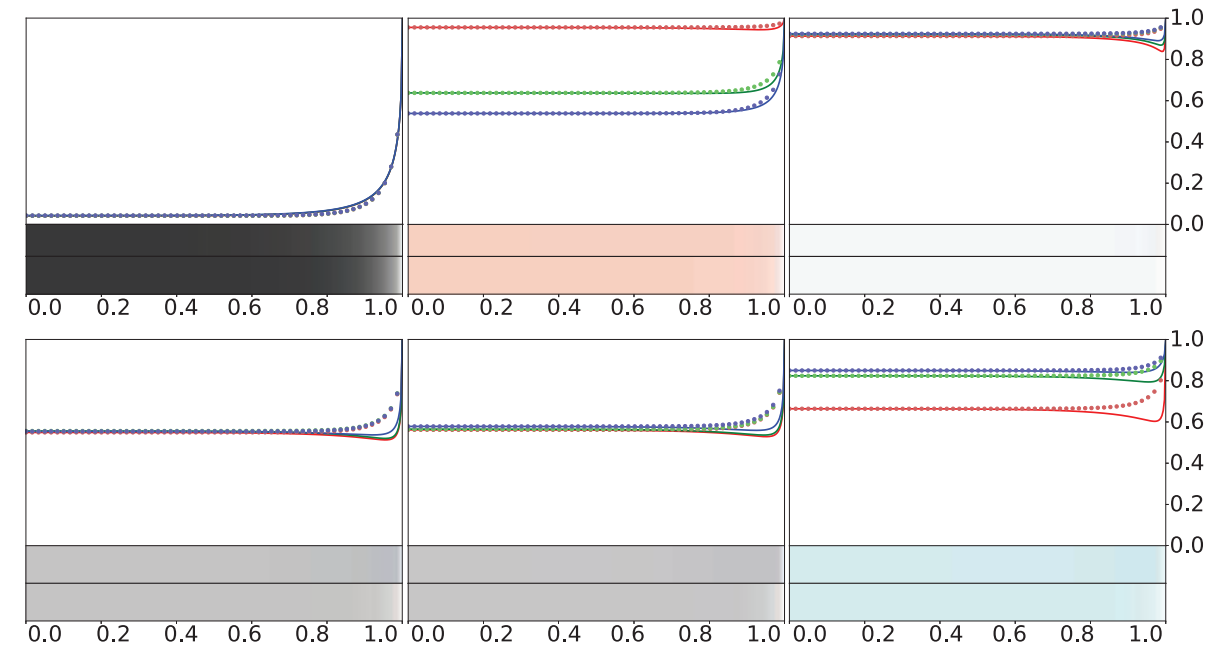$$F_0$$ 也可以用折射率来计算，我们令空气的近似折射率 $$n_1=1$$，然后用 $$n$$ 代替 $$n_2$$ 表示物体的折射率，则有

$F_0 = \left(\frac{n-1}{n+1}\right)^2$

$F(\mathbf{n},\mathbf{l}) \approx F_0 + (F_{90} - F_0)(1-(\mathbf{n}\cdot \mathbf{l})^+)^5$

## 典型的菲涅尔反射率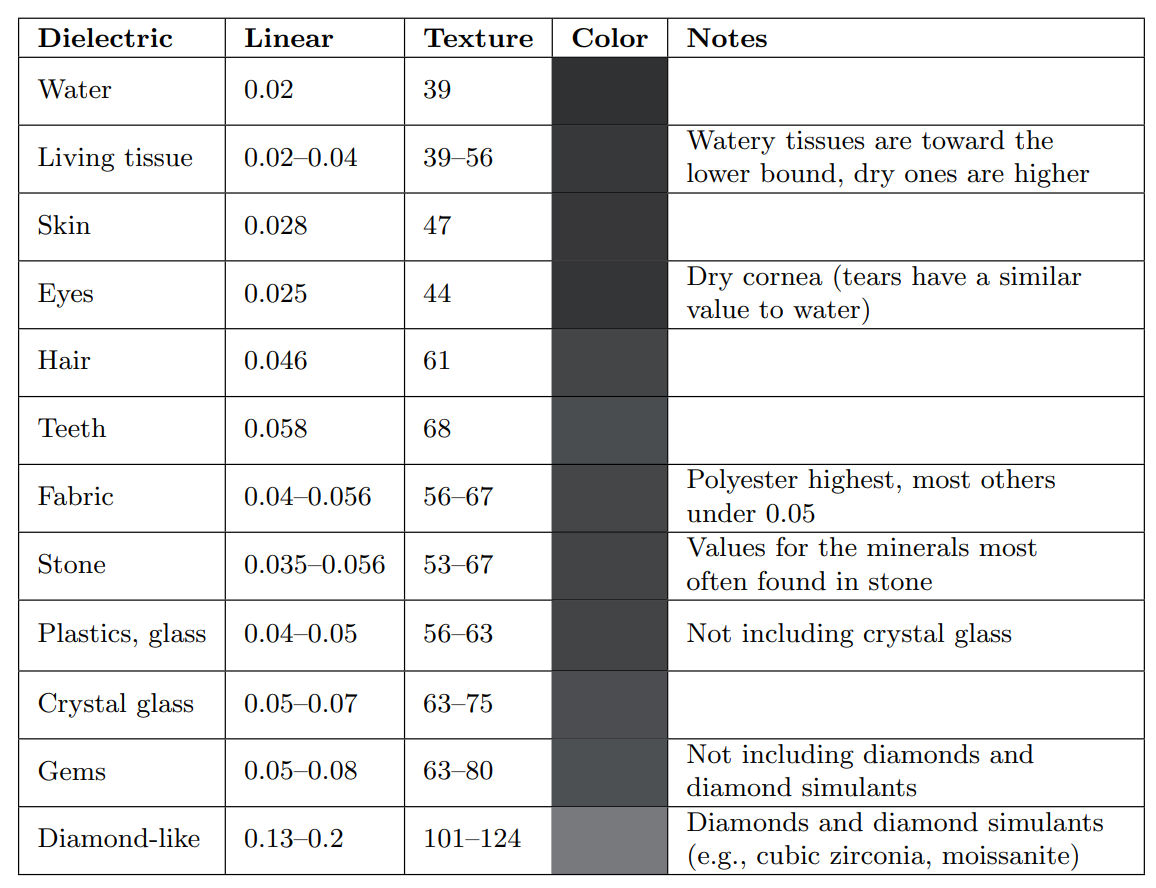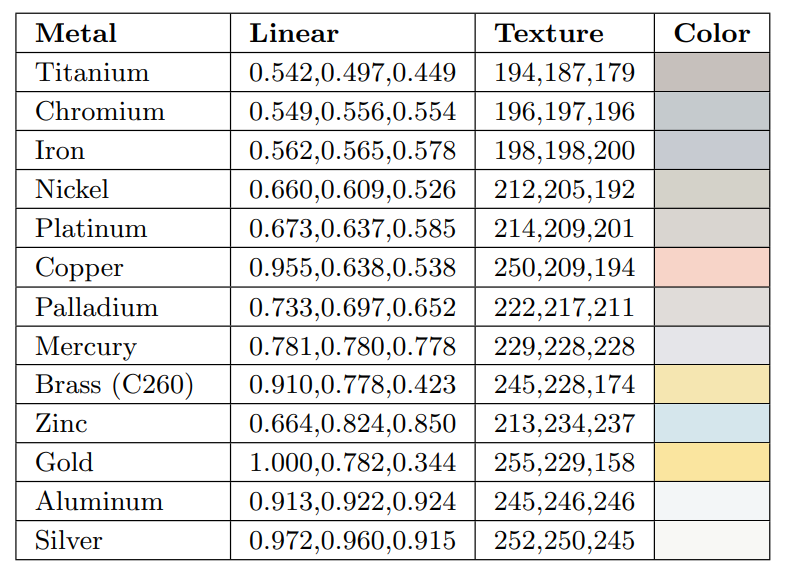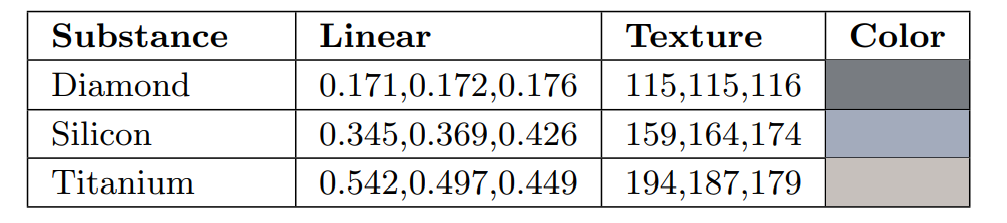$F_0 = \left(\frac{n_1-n_2}{n_1+n_2}\right)^2$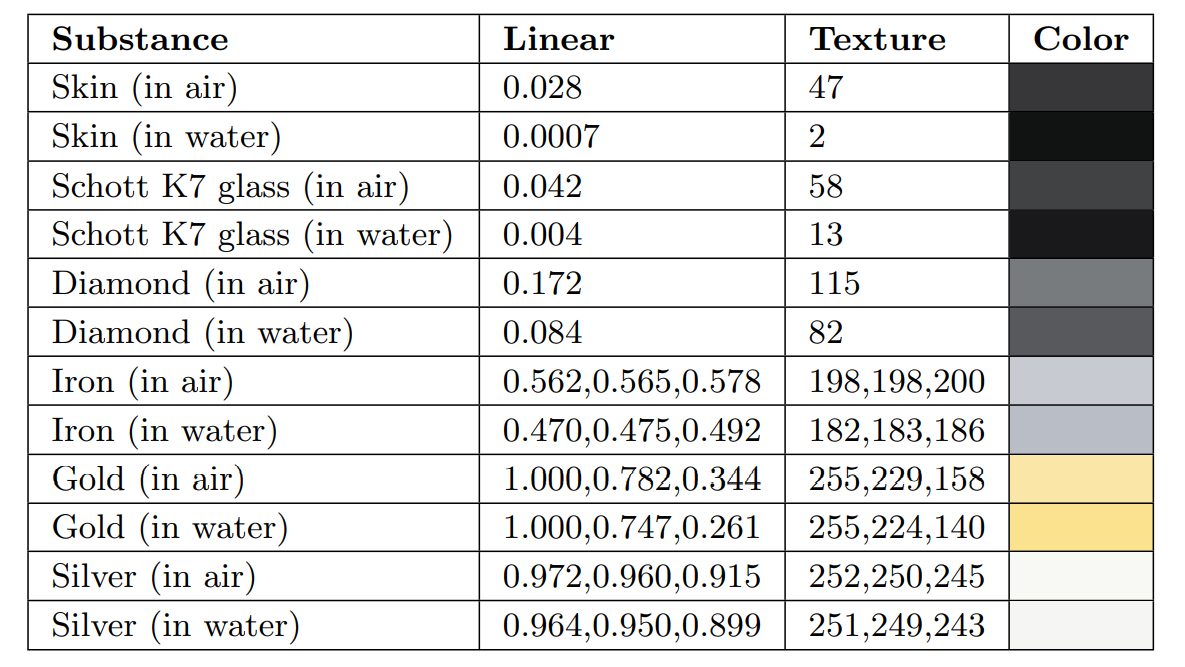## 内反射 Internal Reflection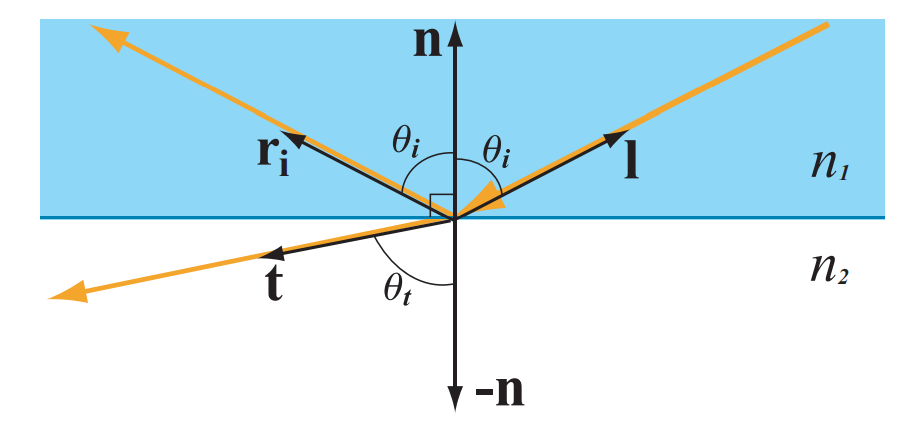$\sin(\theta_t) = \frac{n_1}{n_2} \sin(\theta_i)$

$$n_1>n_2$$ 时，容易发现，当 $$\sin(\theta_i)$$ 还没到达 $$1$$ 时， $$\sin(\theta_t)$$ 就可能到达 $$1$$，此时 $$\theta_i$$ 再增大，将不会发生折射现象，入射光全部反射出去，此现象称为全反射。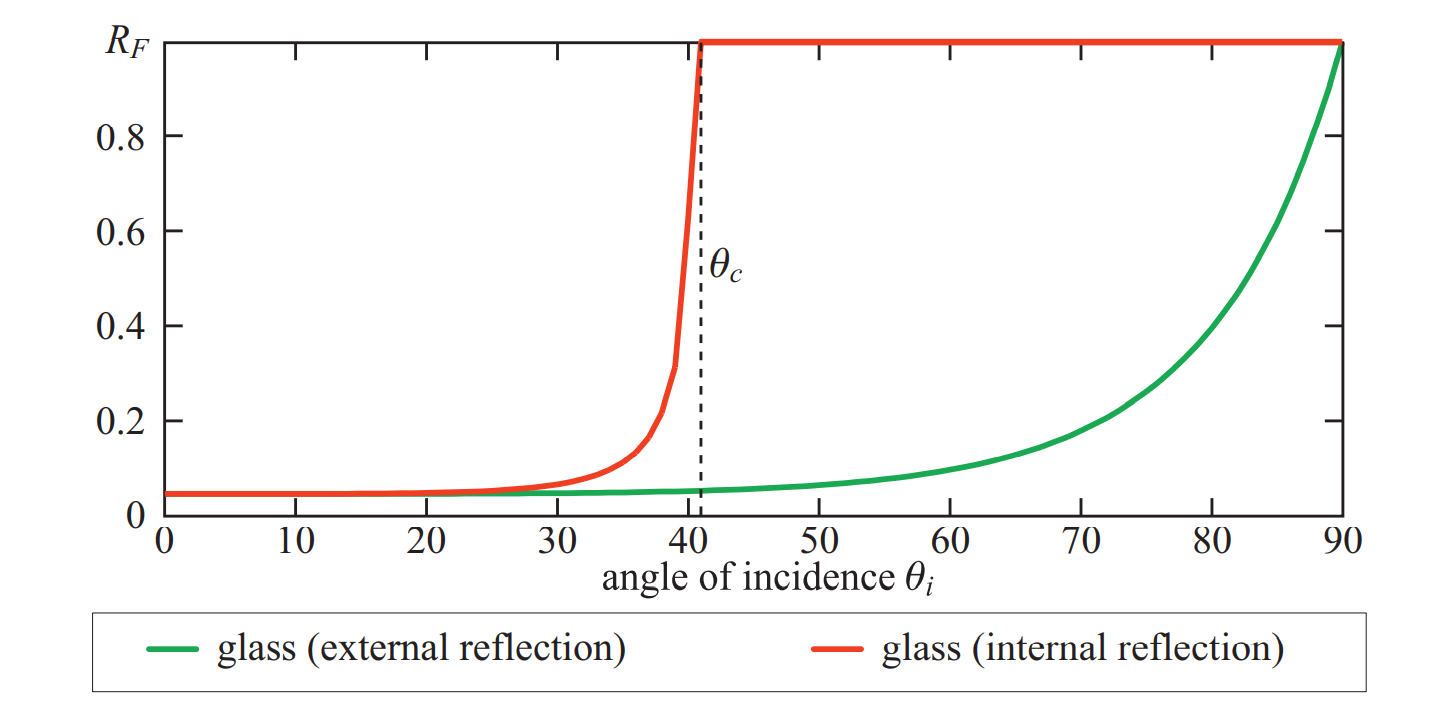$\sin(\theta_c) = \frac{1-\sqrt{F_0}}{1+\sqrt{F_0}}$

$1=\sin(\theta_t) = \frac{n_1}{n_2}\sin(\theta_c)\\ \sin(\theta_c) = \frac{n_2}{n_1}\\ F_0 = \left(\frac{n_1-n_2}{n_1+n_2}\right)^2\\ \sqrt{F_0} = \frac{n_1-n_2}{n_1+n_2}\\ (n_1+n_2)\sqrt{F_0}=n_1-n_2\\ \left(1+\frac{n_2}{n_1}\right)\sqrt{F_0} = 1 - \frac{n_2}{n_1}\\ \sin(\theta_c) = \frac{1-\sqrt{F_0}}{1+\sqrt{F_0}}$

posted @ 2022-10-28 20:21  AE酱  阅读(75)  评论(0编辑  收藏  举报